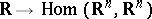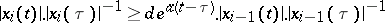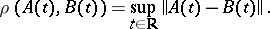# Integral separation condition

(diff) ← Older revision | Latest revision (diff) | Newer revision → (diff)

A condition on a system of linear differential equations(whereis a mappingwith), requiring that the system has solutions,, satisfying for certainthe inequalitiesfor alland all.

The set of systems satisfying the integral separation condition is the interior of the set of continuity of all Lyapunov characteristic exponents (cf. Lyapunov characteristic exponent) in the space of systemswith metricHow to Cite This Entry:
Integral separation condition. Encyclopedia of Mathematics. URL: http://encyclopediaofmath.org/index.php?title=Integral_separation_condition&oldid=11798
This article was adapted from an original article by V.M. Millionshchikov (originator), which appeared in Encyclopedia of Mathematics - ISBN 1402006098. See original article